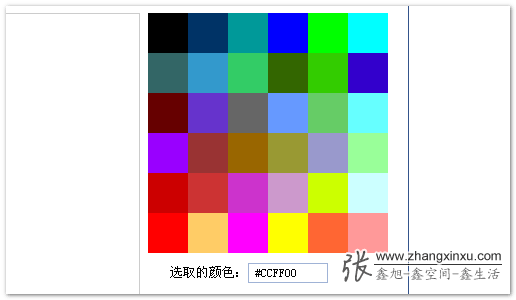# JS HEX十六进制与RGB, HSL颜色的相互转换

2023-09-23 17 0

### JS HEX十六进制与RGB, HSL颜色的相互转换#### 一、关于颜色的表示#### 二、颜色表示方式转换的必要性`<button style="color:#ff3300;" οnclick="javascript:alert(this.style.color);">点击我</button>`#### 三、JavaScript颜色转换的核心

JavaScript颜色转换的核心就是进制间的转换。RGB格式其实就是十进制表示法，所以，十六进制颜色与RGB颜色的转换就是十六进制与十进制之间的转换。

#### 四、JavaScript颜色表示方式的转换的实现

```String.prototype.colorHex = function(){var that = this;//十六进制颜色值的正则表达式var reg = /^#([0-9a-fA-f]{3}|[0-9a-fA-f]{6})\$/;// 如果是rgb颜色表示if (/^(rgb|RGB)/.test(that)) {var aColor = that.replace(/(?:\(|\)|rgb|RGB)*/g, "").split(",");var strHex = "#";for (var i=0; i<aColor.length; i++) {var hex = Number(aColor[i]).toString(16);if (hex.length < 2) {hex = '0' + hex;    }strHex += hex;}if (strHex.length !== 7) {strHex = that;    }return strHex;} else if (reg.test(that)) {var aNum = that.replace(/#/,"").split("");if (aNum.length === 6) {return that;    } else if(aNum.length === 3) {var numHex = "#";for (var i=0; i<aNum.length; i+=1) {numHex += (aNum[i] + aNum[i]);}return numHex;}}return that;
};```

```String.prototype.colorRgb = function(){var sColor = this.toLowerCase();//十六进制颜色值的正则表达式var reg = /^#([0-9a-fA-f]{3}|[0-9a-fA-f]{6})\$/;// 如果是16进制颜色if (sColor && reg.test(sColor)) {if (sColor.length === 4) {var sColorNew = "#";for (var i=1; i<4; i+=1) {sColorNew += sColor.slice(i, i+1).concat(sColor.slice(i, i+1));    }sColor = sColorNew;}//处理六位的颜色值var sColorChange = [];for (var i=1; i<7; i+=2) {sColorChange.push(parseInt("0x"+sColor.slice(i, i+2)));    }return "RGB(" + sColorChange.join(",") + ")";}return sColor;
};```

```var sRgb = "RGB(23, 245, 56)" , sHex = "#34538b";
var sHexColor = sRgb.colorHex();
var sRgbColor = sHex.colorRgb();
```

gold

GRB颜色值：

#17f538

HSL转RGB

```/*** HSL颜色值转换为RGB. * 换算公式改编自 http://en.wikipedia.org/wiki/HSL_color_space.* h, s, 和 l 设定在 [0, 1] 之间* 返回的 r, g, 和 b 在 [0, 255]之间** @param   Number  h       色相* @param   Number  s       饱和度* @param   Number  l       亮度* @return  Array           RGB色值数值*/
function hslToRgb(h, s, l) {var r, g, b;if(s == 0) {r = g = b = l; // achromatic} else {var hue2rgb = function hue2rgb(p, q, t) {if(t < 0) t += 1;if(t > 1) t -= 1;if(t < 1/6) return p + (q - p) * 6 * t;if(t < 1/2) return q;if(t < 2/3) return p + (q - p) * (2/3 - t) * 6;return p;}var q = l < 0.5 ? l * (1 + s) : l + s - l * s;var p = 2 * l - q;r = hue2rgb(p, q, h + 1/3);g = hue2rgb(p, q, h);b = hue2rgb(p, q, h - 1/3);}return [Math.round(r * 255), Math.round(g * 255), Math.round(b * 255)];
}```

RGB转HSL

```/*** RGB 颜色值转换为 HSL.* 转换公式参考自 http://en.wikipedia.org/wiki/HSL_color_space.* r, g, 和 b 需要在 [0, 255] 范围内* 返回的 h, s, 和 l 在 [0, 1] 之间** @param   Number  r       红色色值* @param   Number  g       绿色色值* @param   Number  b       蓝色色值* @return  Array           HSL各值数组*/
function rgbToHsl(r, g, b) {r /= 255, g /= 255, b /= 255;var max = Math.max(r, g, b), min = Math.min(r, g, b);var h, s, l = (max + min) / 2;if (max == min){ h = s = 0; // achromatic} else {var d = max - min;s = l > 0.5 ? d / (2 - max - min) : d / (max + min);switch(max) {case r: h = (g - b) / d + (g < b ? 6 : 0); break;case g: h = (b - r) / d + 2; break;case b: h = (r - g) / d + 4; break;}h /= 6;}return [h, s, l];
}```

H指Hue(色调)。 0(或360)表示红色，120表示绿色，240表示蓝色，当然可取其他数值来确定其它颜色；
S指Saturation(饱和度)。 取值为0%到100%之间的值；
L指Lightness(亮度)。 取值为0%到100%之间的值；

```var colorToRgb = function (color) {var div = document.createElement('div');div.style.backgroundColor = color;document.body.appendChild(div);var c = window.getComputedStyle(div).backgroundColor;    document.body.removeChild(div);return c;
};```